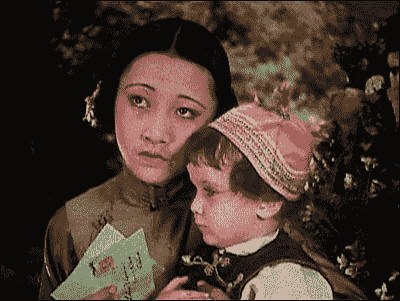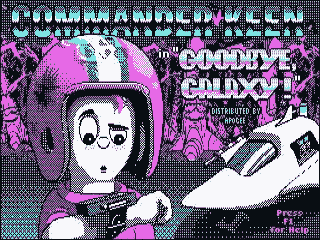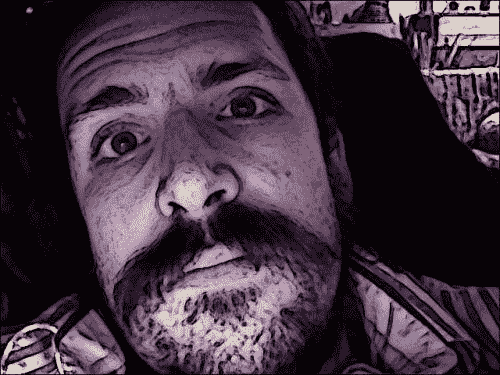# 创建模块

CaptureManagerWindowManager类一样，我们的过滤器应可在 Cameo 外部重用。 因此，我们应该将过滤器分成各自的 Python 模块或文件。

import cv2
import numpy
import utils


import cv2
import numpy
import scipy.interpolate


# 通道混合 – 在 Technicolor 中查看

dst.b = funcB(src.b, src.g, src.r)
dst.g = funcG(src.b, src.g, src.r)
dst.r = funcR(src.b, src.g, src.r)


• RC（红色，青色）：请注意，红色和青色可以混合成灰色。 该色彩空间类似于 Technicolor 流程 2 和 CGA 调色板 3。
• RGV（红色，绿色，值）：请注意，红色和绿色不能混合成灰色。 因此，我们还需要指定值或白度。 此色彩空间类似于 Technicolor 流程 1。
• CMV（青色，洋红色，值）：请注意，青色和洋红色不能混合以产生灰色。 因此，我们还需要指定值或白度。 此颜色空间类似于 CGA 调色板 1。## 模拟 RC 色彩空间

RC 色彩空间易于在 BGR 中进行模拟。 蓝色和绿色可以混合成青色。 通过平均 B 和 G 通道并将结果存储在 B 和 G 中，我们有效地将这两个通道合为一个 C。为了支持这种效果，让我们向filters.py添加以下函数：

def recolorRC(src, dst):
"""Simulate conversion from BGR to RC (red, cyan).

The source and destination images must both be in BGR format.

Blues and greens are replaced with cyans.

Pseudocode:
dst.b = dst.g = 0.5 * (src.b + src.g)
dst.r = src.r

"""
b, g, r = cv2.split(src)
cv2.addWeighted(b, 0.5, g, 0.5, 0, b)
cv2.merge((b, b, r), dst)


1. 使用split()，我们将源图像的通道提取为一维数组。 以这种格式放置数据后，我们可以编写清晰，简单的通道混合代码。
2. 使用addWeighted()，我们用 B 和 G 的平均值替换 B 通道的值。addWeighted()的参数（按顺序）是第一个源数组，一个权重应用于第一个源数组，第二个源数组， 应用于第二个源数组的权重，添加到结果中的常量以及一个目标数组。
3. 使用merge()，我们用修改后的通道替换目标图像中的值。 请注意，我们两次使用b作为参数，因为我们希望目标的 B 和 G 通道相等。

## 模拟 RGV 色彩空间

def recolorRGV(src, dst):
"""Simulate conversion from BGR to RGV (red, green, value).

The source and destination images must both be in BGR format.

Blues are desaturated.

Pseudocode:
dst.b = min(src.b, src.g, src.r)
dst.g = src.g
dst.r = src.r

"""
b, g, r = cv2.split(src)
cv2.min(b, g, b)
cv2.min(b, r, b)
cv2.merge((b, g, r), dst)


min()函数计算前两个参数的每个元素的最小值，并将它们写入第三个参数。

## 模拟 CMV 颜色空间

def recolorCMV(src, dst):
"""Simulate conversion from BGR to CMV (cyan, magenta, value).

The source and destination images must both be in BGR format.

Yellows are desaturated.

Pseudocode:
dst.b = max(src.b, src.g, src.r)
dst.g = src.g
dst.r = src.r

"""
b, g, r = cv2.split(src)
cv2.max(b, g, b)
cv2.max(b, r, b)
cv2.merge((b, g, r), dst)


max()函数计算前两个参数的每个元素的最大值，并将它们写入第三个参数。

# 曲线 – 弯曲色彩空间

dst.b = funcB(src.b) where funcB interpolates pointsB
dst.g = funcG(src.g) where funcG interpolates pointsG
dst.r = funcR(src.r) where funcR interpolates pointsR


## 绘制曲线

def createCurveFunc(points):
"""Return a function derived from control points."""
if points is None:
return None
numPoints = len(points)
if numPoints < 2:
return None
xs, ys = zip(*points)
if numPoints < 4:
kind = 'linear'
else:
kind = 'cubic'
return scipy.interpolate.interp1d(xs, ys, kind,
bounds_error = False)


## 缓存和应用曲线

def createLookupArray(func, length = 256):
"""Return a lookup for whole-number inputs to a function.

The lookup values are clamped to [0, length - 1].

"""
if func is None:
return None
lookupArray = numpy.empty(length)
i = 0
while i < length:
func_i = func(i)
lookupArray[i] = min(max(0, func_i), length - 1)
i += 1
return lookupArray

def applyLookupArray(lookupArray, src, dst):
"""Map a source to a destination using a lookup."""
if lookupArray is None:
return
dst[:] = lookupArray[src]


def createCompositeFunc(func0, func1):
"""Return a composite of two functions."""
if func0 is None:
return func1
if func1 is None:
return func0
return lambda x: func0(func1(x))


createCompositeFunc()中的方法仅限于每个都带有单个参数的输入函数。 参数必须是兼容类型。 请注意，使用 Python 的lambda关键字创建匿名函数。

def createFlatView(array):
"""Return a 1D view of an array of any dimensionality."""
flatView = array.view()
flatView.shape = array.size
return flatView


createFlatView()中的方法适用于具有任意数量通道的图像。 因此，当我们希望所有通道都相同时，它可以抽象出灰度图像和彩色图像之间的差异。

## 设计面向对象的曲线过滤器

• VFuncFilter：这是一个用函数实例化的类，以后可以使用apply()将其应用于图像。 该函数适用​​于灰度图像的 V（值）通道或彩色图像的所有通道。
• VcurveFilter：这是VFuncFilter的子类。 而不是使用函数实例化，而是使用一组控制点实例化，这些控制点在内部用于创建曲线函数。
• BGRFuncFilter：这是一个用最多四个函数实例化的类，以后可以使用apply()将其应用于 BGR 图像。 这些函数之一适用于所有通道，其他三个函数分别适用于单个通道。 首先应用整体函数，然后再应用每通道函数。
• BGRCurveFilter：这是BGRFuncFilter的子类。 而不是用四个函数实例化，而是用四组控制点实例化，这些控制点在内部用于创建曲线函数。

class VFuncFilter(object):
"""A filter that applies a function to V (or all of BGR)."""

def __init__(self, vFunc = None, dtype = numpy.uint8):
length = numpy.iinfo(dtype).max + 1
self._vLookupArray = utils.createLookupArray(vFunc, length)

def apply(self, src, dst):
"""Apply the filter with a BGR or gray source/destination."""
srcFlatView = utils.flatView(src)
dstFlatView = utils.flatView(dst)
utils.applyLookupArray(self._vLookupArray, srcFlatView,
dstFlatView)

class VCurveFilter(VFuncFilter):
"""A filter that applies a curve to V (or all of BGR)."""

def __init__(self, vPoints, dtype = numpy.uint8):
VFuncFilter.__init__(self, utils.createCurveFunc(vPoints),
dtype)


class BGRFuncFilter(object):
"""A filter that applies different functions to each of BGR."""

def __init__(self, vFunc = None, bFunc = None, gFunc = None,
rFunc = None, dtype = numpy.uint8):
length = numpy.iinfo(dtype).max + 1
self._bLookupArray = utils.createLookupArray(
utils.createCompositeFunc(bFunc, vFunc), length)
self._gLookupArray = utils.createLookupArray(
utils.createCompositeFunc(gFunc, vFunc), length)
self._rLookupArray = utils.createLookupArray(
utils.createCompositeFunc(rFunc, vFunc), length)

def apply(self, src, dst):
"""Apply the filter with a BGR source/destination."""
b, g, r = cv2.split(src)
utils.applyLookupArray(self._bLookupArray, b, b)
utils.applyLookupArray(self._gLookupArray, g, g)
utils.applyLookupArray(self._rLookupArray, r, r)
cv2.merge([b, g, r], dst)

class BGRCurveFilter(BGRFuncFilter):
"""A filter that applies different curves to each of BGR."""

def __init__(self, vPoints = None, bPoints = None,
gPoints = None, rPoints = None, dtype = numpy.uint8):
BGRFuncFilter.__init__(self,
utils.createCurveFunc(vPoints),
utils.createCurveFunc(bPoints),
utils.createCurveFunc(gPoints),
utils.createCurveFunc(rPoints), dtype)


## 模拟摄影胶片

• 柯达 Portra，专为肖像和婚礼而设计的胶卷系列
• 通用电影系列富士 Provia
• 富士 Velvia，针对风景优化的电影系列
• 交叉处理，一种非标准的胶片处理技术，有时用于在时装和乐队摄影中产生低劣的外观

Portra，Provia 和 Velvia 效果应产生外观正常的图像。 除了前后比较之外，效果应该不明显。

### 模拟柯达 Portra

Portra 具有较宽的高光范围，倾向于暖色（琥珀色），而阴影较冷（更蓝）。 作为人像电影，它倾向于使人们的肤色更白皙。 而且，它会夸大某些常见的衣服颜色，例如乳白色（例如婚纱）和深蓝色（例如西装或牛仔裤）。 让我们将 Portra 过滤器的此实现添加到filters.py

class BGRPortraCurveFilter(BGRCurveFilter):
"""A filter that applies Portra-like curves to BGR."""

def __init__(self, dtype = numpy.uint8):
BGRCurveFilter.__init__(
self,
vPoints = [(0,0),(23,20),(157,173),(255,255)],
bPoints = [(0,0),(41,46),(231,228),(255,255)],
gPoints = [(0,0),(52,47),(189,196),(255,255)],
rPoints = [(0,0),(69,69),(213,218),(255,255)],
dtype = dtype)


### 模拟富士 Provia

class BGRProviaCurveFilter(BGRCurveFilter):
"""A filter that applies Provia-like curves to BGR."""

def __init__(self, dtype = numpy.uint8):
BGRCurveFilter.__init__(
self,
bPoints = [(0,0),(35,25),(205,227),(255,255)],
gPoints = [(0,0),(27,21),(196,207),(255,255)],
rPoints = [(0,0),(59,54),(202,210),(255,255)],
dtype = dtype)


### 模拟富士 Velvia

Velvia 具有深阴影和鲜艳的色彩。 它通常可以在白天产生蔚蓝的天空，在日落时产生深红色的云。 效果很难模拟，但是这是我们可以添加到filters.py的尝试：

class BGRVelviaCurveFilter(BGRCurveFilter):
"""A filter that applies Velvia-like curves to BGR."""

def __init__(self, dtype = numpy.uint8):
BGRCurveFilter.__init__(
self,
vPoints = [(0,0),(128,118),(221,215),(255,255)],
bPoints = [(0,0),(25,21),(122,153),(165,206),(255,255)],
gPoints = [(0,0),(25,21),(95,102),(181,208),(255,255)],
rPoints = [(0,0),(41,28),(183,209),(255,255)],
dtype = dtype)


### 模拟交叉处理

class BGRCrossProcessCurveFilter(BGRCurveFilter):
"""A filter that applies cross-process-like curves to BGR."""

def __init__(self, dtype = numpy.uint8):
BGRCurveFilter.__init__(
self,
bPoints = [(0,20),(255,235)],
gPoints = [(0,0),(56,39),(208,226),(255,255)],
rPoints = [(0,0),(56,22),(211,255),(255,255)],
dtype = dtype)


# 突出显示边缘

OpenCV 提供了许多边缘过滤器，包括Laplacian()Sobel()Scharr()。 这些过滤器应该将非边缘区域变成黑色，而将边缘区域变成白色或饱和色。 但是，它们易于将噪声误识别为边缘。 可以通过在尝试查找边缘之前对图像进行模糊处理来缓解此缺陷。 OpenCV 还提供了许多模糊过滤器，包括blur()（简单平均值），medianBlur()GaussianBlur()。 边缘查找和模糊过滤器的参数有所不同，但始终包含ksize，这是一个奇数，代表过滤器核的宽度和高度（以像素为单位）。

### 注意

def strokeEdges(src, dst, blurKsize = 7, edgeKsize = 5):
if blurKsize >= 3:
blurredSrc = cv2.medianBlur(src, blurKsize)
graySrc = cv2.cvtColor(blurredSrc, cv2.COLOR_BGR2GRAY)
else:
graySrc = cv2.cvtColor(src, cv2.COLOR_BGR2GRAY)
cv2.Laplacian(graySrc, cv2.cv.CV_8U, graySrc, ksize = edgeKsize)
normalizedInverseAlpha = (1.0 / 255) * (255 - graySrc)
channels = cv2.split(src)
for channel in channels:
channel[:] = channel * normalizedInverseAlpha
cv2.merge(channels, dst)


# 自定义核 – 令人费解

OpenCV 提供了非常通用的函数filter2D()和，该函数应用了我们指定的任何核或卷积矩阵。 要了解如何使用此函数，让我们首先学习卷积矩阵的格式。 它是一个二维数组，具有奇数行和列。 中心元素对应于关注像素，其他元素对应于该像素的邻居。 每个元素都包含一个整数或浮点值，该值是应用于输入像素值的权重。 考虑以下示例：

kernel = numpy.array([[-1, -1, -1],
[-1,  9, -1],
[-1, -1, -1]])


cv2.filter2D(src, -1, kernel, dst)


### 注意

class VConvolutionFilter(object):
"""A filter that applies a convolution to V (or all of BGR)."""

def __init__(self, kernel):
self._kernel = kernel

def apply(self, src, dst):
"""Apply the filter with a BGR or gray source/destination."""
cv2.filter2D(src, -1, self._kernel, dst)

class SharpenFilter(VConvolutionFilter):
"""A sharpen filter with a 1-pixel radius."""

def __init__(self):
kernel = numpy.array([[-1, -1, -1],
[-1,  9, -1],
[-1, -1, -1]])
VConvolutionFilter.__init__(self, kernel)


class FindEdgesFilter(VConvolutionFilter):
"""An edge-finding filter with a 1-pixel radius."""

def __init__(self):
kernel = numpy.array([[-1, -1, -1],
[-1,  8, -1],
[-1, -1, -1]])
VConvolutionFilter.__init__(self, kernel)


class BlurFilter(VConvolutionFilter):
"""A blur filter with a 2-pixel radius."""

def __init__(self):
kernel = numpy.array([[0.04, 0.04, 0.04, 0.04, 0.04],
[0.04, 0.04, 0.04, 0.04, 0.04],
[0.04, 0.04, 0.04, 0.04, 0.04],
[0.04, 0.04, 0.04, 0.04, 0.04],
[0.04, 0.04, 0.04, 0.04, 0.04]])
VConvolutionFilter.__init__(self, kernel)


class EmbossFilter(VConvolutionFilter):
"""An emboss filter with a 1-pixel radius."""

def __init__(self):
kernel = numpy.array([[-2, -1, 0],
[-1,  1, 1],
[ 0,  1, 2]])
VConvolutionFilter.__init__(self, kernel)


# 修改应用

import cv2
import filters
from managers import WindowManager, CaptureManager

class Cameo(object):

def __init__(self):
self._windowManager = WindowManager('Cameo',
self.onKeypress)
self._captureManager = CaptureManager(
cv2.VideoCapture(0), self._windowManager, True)
self._curveFilter = filters.BGRPortraCurveFilter()

def run(self):
"""Run the main loop."""
self._windowManager.createWindow()
while self._windowManager.isWindowCreated:
self._captureManager.enterFrame()
frame = self._captureManager.frame

# TODO: Track faces (Chapter 3).

filters.strokeEdges(frame, frame)
self._curveFilter.apply(frame, frame)

self._captureManager.exitFrame()
self._windowManager.processEvents()

# ... The rest is the same as in Chapter 2.